# Plotting datetime charts

PyGMT accepts a variety of datetime objects to plot data and create charts. Aside from the built-in Python `datetime` object, PyGMT supports input using ISO formatted strings, `pandas`, `xarray`, as well as `numpy`. These data types can be used to plot specific points as well as get passed into the `region` parameter to create a range of the data on an axis.

The following examples will demonstrate how to create plots using the different datetime objects.

```import datetime

import numpy as np
import pandas as pd
import pygmt
import xarray as xr
```

## Using Python’s `datetime`

In this example, Python’s built-in `datetime` module is used to create data points stored in list `x`. Additionally, dates are passed into the `region` parameter in the format `(x_start, x_end, y_start, y_end)`, where the date range is plotted on the x-axis. An additional notable parameter is `style`, where it’s specified that data points are to be plotted in an X shape with a size of 0.3 centimeters.

```x = [
datetime.date(2010, 6, 1),
datetime.date(2011, 6, 1),
datetime.date(2012, 6, 1),
datetime.date(2013, 6, 1),
]
y = [1, 2, 3, 5]

fig = pygmt.Figure()
fig.plot(
projection="X10c/5c",
region=[datetime.date(2010, 1, 1), datetime.date(2014, 12, 1), 0, 6],
frame=["WSen", "afg"],
x=x,
y=y,
style="x0.3c",
pen="1p",
)
fig.show()
```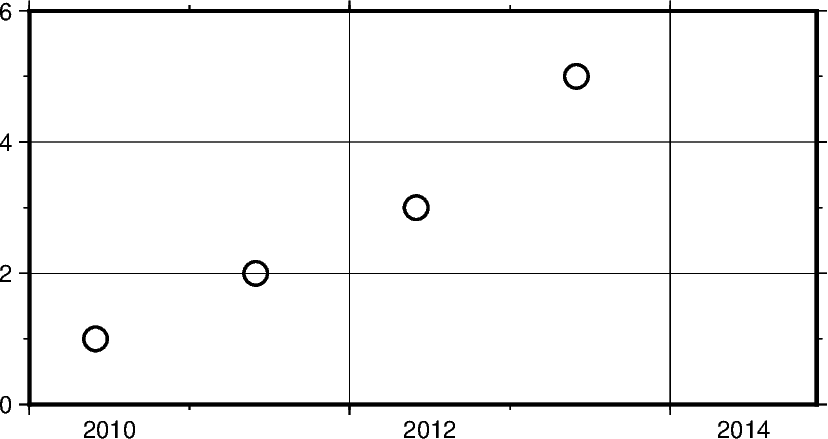Out:

```<IPython.core.display.Image object>
```

In addition to specifying the date, `datetime` supports the exact time at which the data points were recorded. Using `datetime.datetime` the `region` parameter as well as data points can be created with both date and time information.

Some notable differences to the previous example include

• Modifying `frame` to only include West (left) and South (bottom) borders, and removing grid lines

• Using circles to plot data points defined through `c` in `style` parameter

```x = [
datetime.datetime(2021, 1, 1, 3, 45, 1),
datetime.datetime(2021, 1, 1, 6, 15, 1),
datetime.datetime(2021, 1, 1, 13, 30, 1),
datetime.datetime(2021, 1, 1, 20, 30, 1),
]
y = [5, 3, 1, 2]

fig = pygmt.Figure()
fig.plot(
projection="X10c/5c",
region=[
datetime.datetime(2021, 1, 1, 0, 0, 0),
datetime.datetime(2021, 1, 2, 0, 0, 0),
0,
6,
],
frame=["WS", "af"],
x=x,
y=y,
style="c0.4c",
pen="1p",
color="blue",
)
fig.show()
```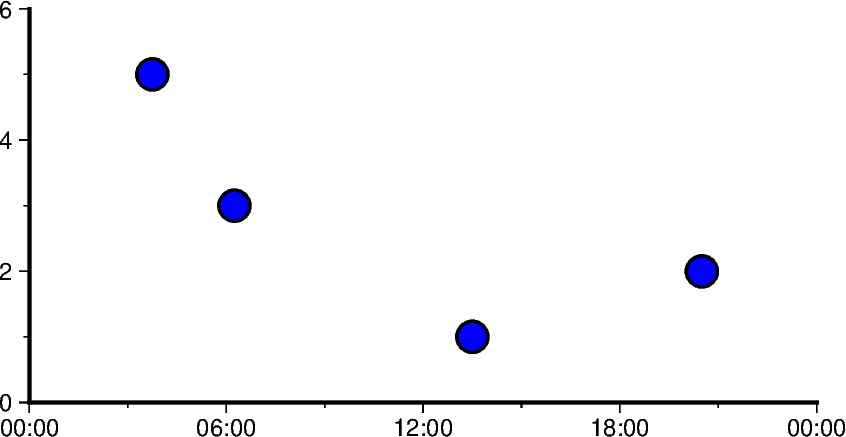Out:

```<IPython.core.display.Image object>
```

## Using ISO Format

In addition to Python’s `datetime` library, PyGMT also supports passing times in ISO format. Basic ISO strings are formatted as `YYYY-MM-DD` with each `-` delineated section marking the four digit year value, two digit month value, and two digit day value respectively.

When including time of day into ISO strings, the `T` character is used, as can be seen in the following example. This character is immediately followed by a string formatted as `hh:mm:ss` where each `:` delineated section marking the two digit hour value, two digit minute value, and two digit second value respectively. The figure in the following example is plotted over a horizontal range of one year from 1/1/2016 to 1/1/2017.

```x = ["2016-02-01", "2016-06-04T14", "2016-10-04T00:00:15", "2016-12-01T05:00:15"]
y = [1, 3, 5, 2]
fig = pygmt.Figure()
fig.plot(
projection="X10c/5c",
region=["2016-01-01", "2017-01-1", 0, 6],
frame=["WSen", "afg"],
x=x,
y=y,
style="a0.45c",
pen="1p",
color="dodgerblue",
)
fig.show()
```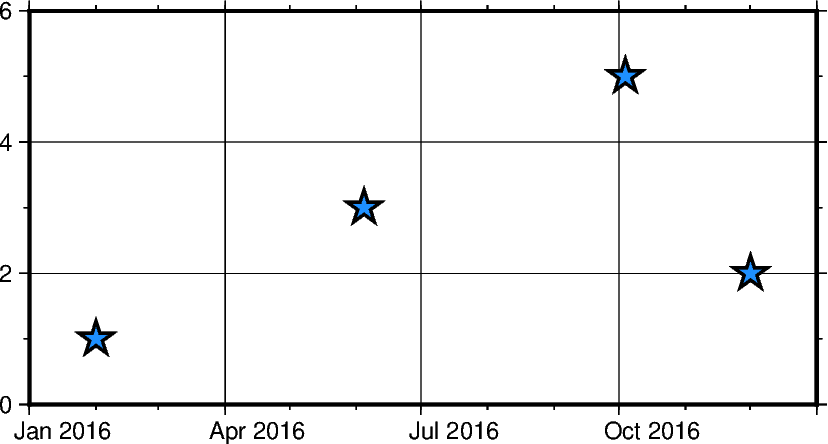Out:

```<IPython.core.display.Image object>
```

## Mixing and matching Python `datetime` and ISO dates

The following example provides context on how both `datetime` and ISO date data can be plotted using PyGMT. This can be helpful when dates and times are coming from different sources, meaning conversions do not need to take place between ISO and datetime in order to create valid plots.

```x = ["2020-02-01", "2020-06-04", "2020-10-04", datetime.datetime(2021, 1, 15)]
y = [1.3, 2.2, 4.1, 3]
fig = pygmt.Figure()
fig.plot(
projection="X10c/5c",
region=[datetime.datetime(2020, 1, 1), datetime.datetime(2021, 3, 1), 0, 6],
frame=["WSen", "afg"],
x=x,
y=y,
style="i0.4c",
pen="1p",
color="yellow",
)
fig.show()
```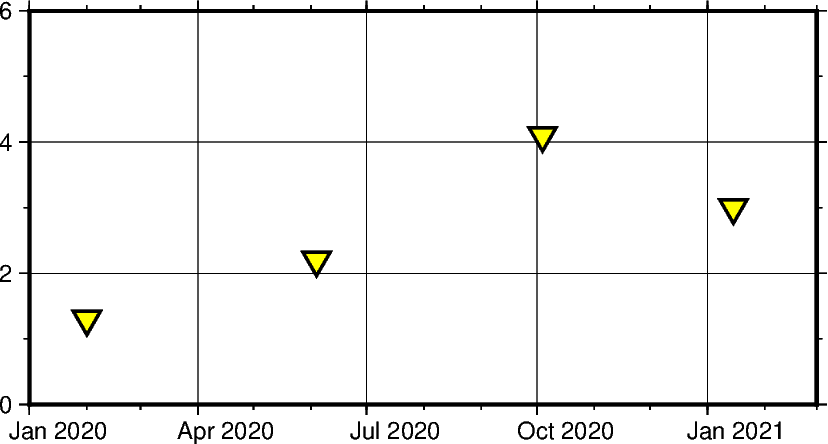Out:

```<IPython.core.display.Image object>
```

## Using `pandas.date_range`

In the following example, `pandas.date_range` produces a list of `pandas.DatetimeIndex` objects, which gets is used to pass date data to the PyGMT figure. Specifically `x` contains 7 different `pandas.DatetimeIndex` objects, with the number being manipulated by the `periods` parameter. Each period begins at the start of a business quarter as denoted by BQS when passed to the `periods` parameter. The initial date is the first argument that is passed to `pandas.date_range` and it marks the first data point in the list `x` that will be plotted.

```x = pd.date_range("2018-03-01", periods=7, freq="BQS")
y = [4, 5, 6, 8, 6, 3, 5]

fig = pygmt.Figure()
fig.plot(
projection="X10c/10c",
region=[datetime.datetime(2017, 12, 31), datetime.datetime(2019, 12, 31), 0, 10],
frame=["WSen", "ag"],
x=x,
y=y,
style="i0.4c",
pen="1p",
color="purple",
)
fig.show()
```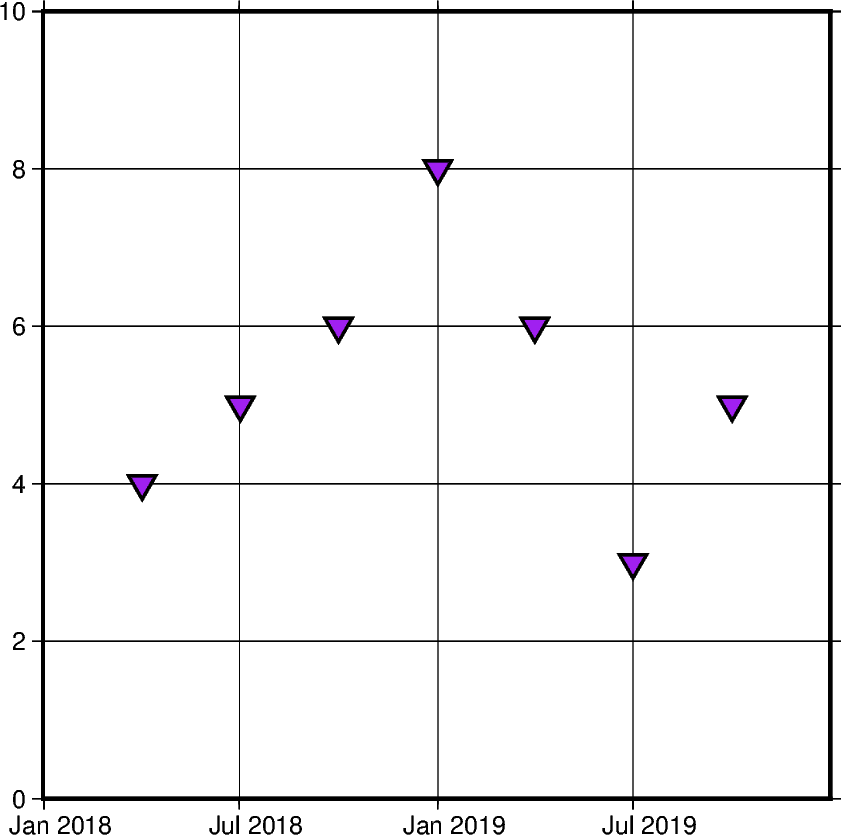Out:

```<IPython.core.display.Image object>
```

## Using `xarray.DataArray`

In this example, instead of using a `pandas.date_range`, `x` is initialized as a list of `xarray.DataArray` objects. This object provides a wrapper around regular PyData formats. It also allows the data to have labeled dimensions while supporting operations that use various pieces of metadata.The following code uses `pandas.date_range` object to fill the DataArray with data, but this is not essential for the creation of a valid DataArray.

```x = xr.DataArray(data=pd.date_range(start="2020-01-01", periods=4, freq="Q"))
y = [4, 7, 5, 6]

fig = pygmt.Figure()
fig.plot(
projection="X10c/10c",
region=[datetime.datetime(2020, 1, 1), datetime.datetime(2021, 4, 1), 0, 10],
frame=["WSen", "ag"],
x=x,
y=y,
style="n0.4c",
pen="1p",
color="red",
)
fig.show()
```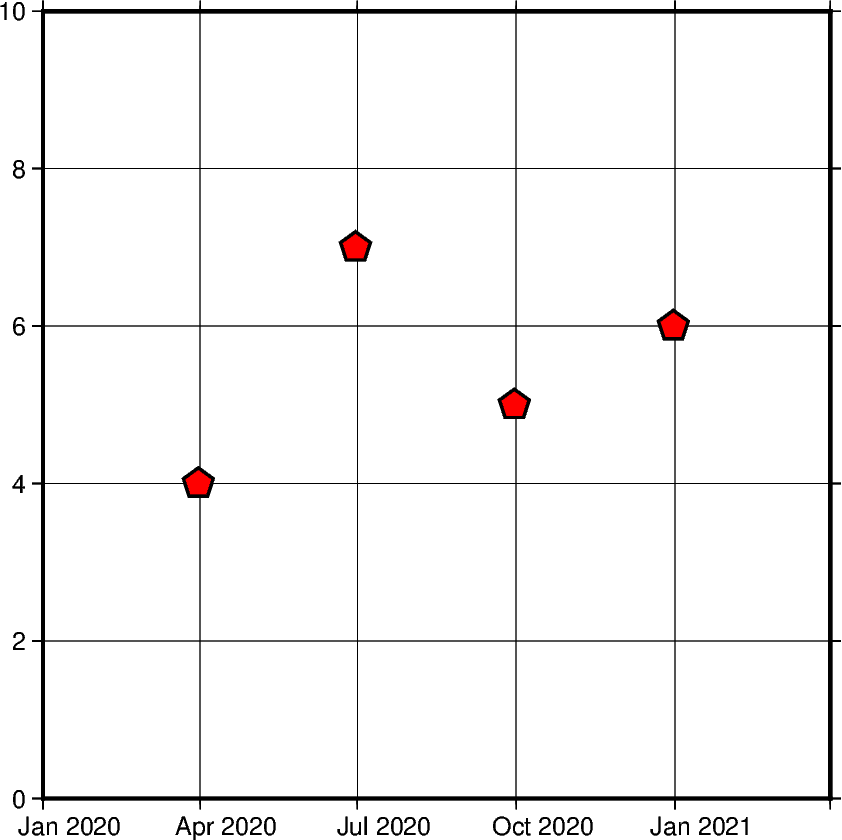Out:

```<IPython.core.display.Image object>
```

## Using `numpy.datetime64`

In this example, instead of using a `pd.date_range`, `x` is initialized as an `np.array` object. Similar to `xarray.DataArray` this wraps the dataset before passing it as a parameter. However, `np.array` objects use less memory and allow developers to specify datatypes.

```x = np.array(["2010-06-01", "2011-06-01T12", "2012-01-01T12:34:56"], dtype="datetime64")
y = [2, 7, 5]

fig = pygmt.Figure()
fig.plot(
projection="X10c/10c",
region=[datetime.datetime(2010, 1, 1), datetime.datetime(2012, 6, 1), 0, 10],
frame=["WS", "ag"],
x=x,
y=y,
style="s0.5c",
pen="1p",
color="blue",
)
fig.show()
```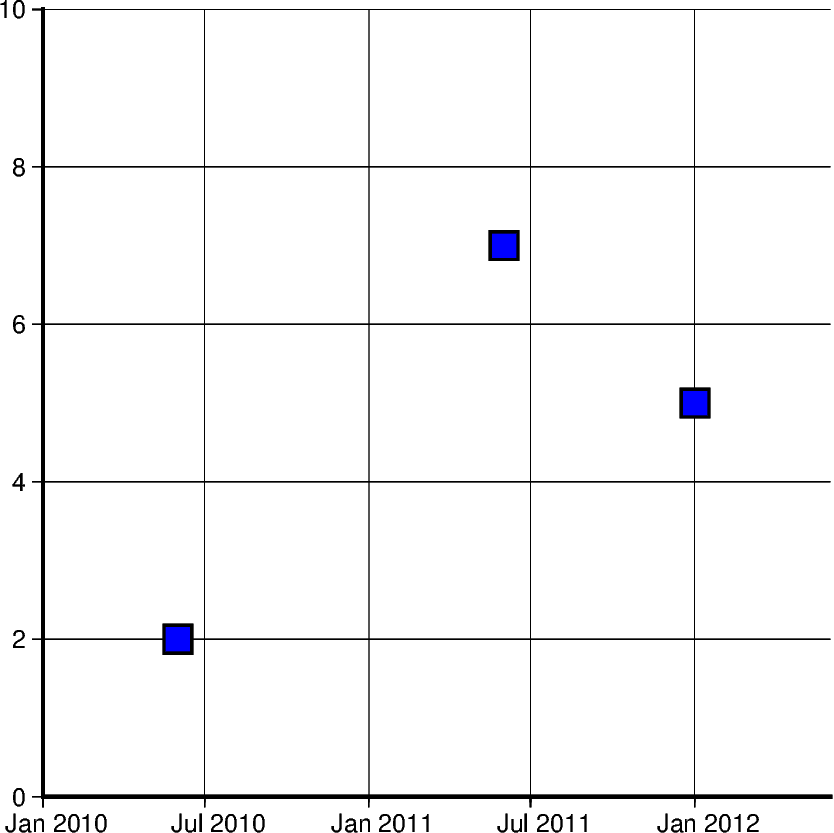Out:

```<IPython.core.display.Image object>
```

## Generating an automatic region

Another way of creating charts involving datetime data can be done by automatically generating the region of the plot. This can be done by passing the dataframe to `pygmt.info`, which will find maximum and minimum values for each column and create a list that could be passed as region. Additionally, the `spacing` argument can be passed to increase the range past the maximum and minimum data points.

```data = [
["20200712", 1000],
["20200714", 1235],
["20200716", 1336],
["20200719", 1176],
["20200721", 1573],
["20200724", 1893],
["20200729", 1634],
]
df = pd.DataFrame(data, columns=["Date", "Score"])
df.Date = pd.to_datetime(df["Date"], format="%Y%m%d")

fig = pygmt.Figure()
region = pygmt.info(
data=df[["Date", "Score"]], per_column=True, spacing=(700, 700), coltypes="T"
)

fig.plot(
region=region,
projection="X15c/10c",
frame=["WSen", "afg"],
x=df.Date,
y=df.Score,
style="c0.4c",
pen="1p",
color="green3",
)

fig.show()
```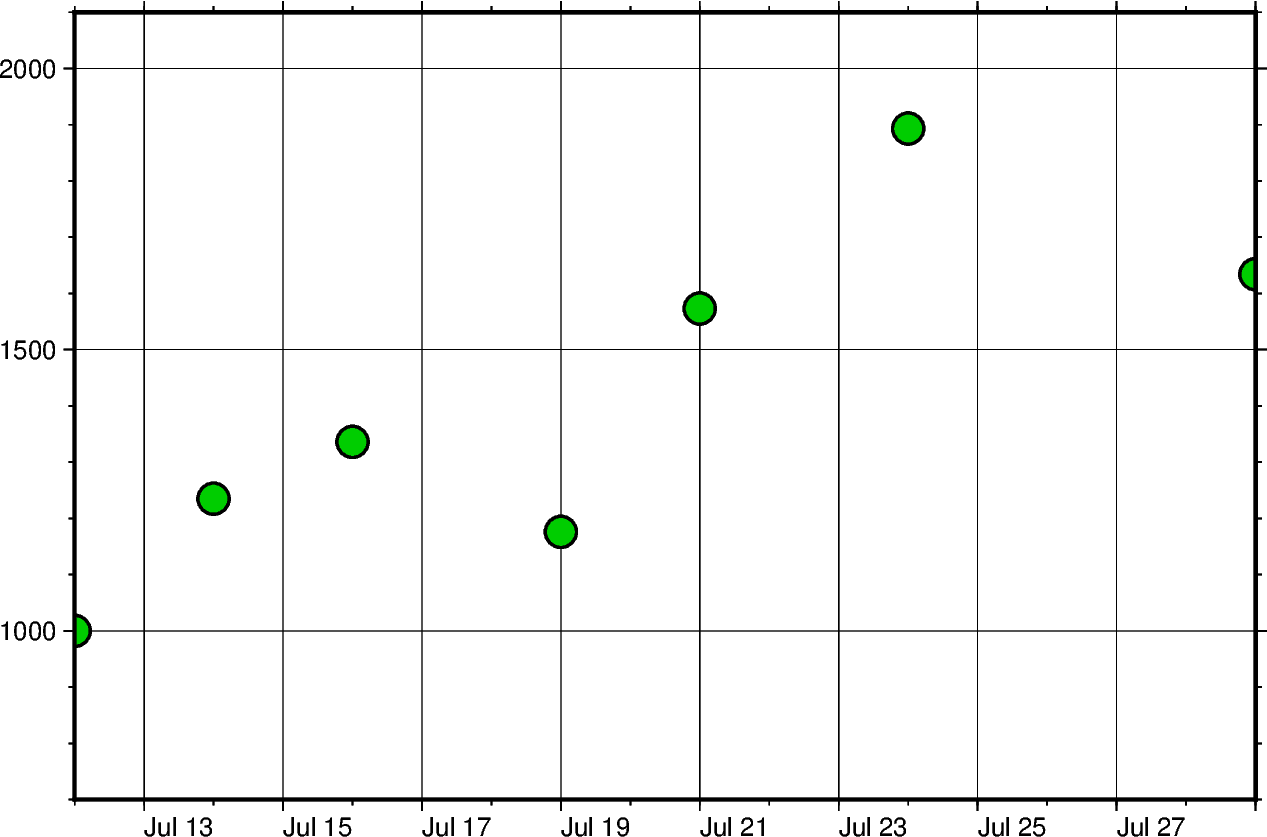Out:

```<IPython.core.display.Image object>
```

## Setting Primary and Secondary Time Axes

This example focuses on labeling the axes and setting intervals at which the labels are expected to appear. All of these modifications are added to the `frame` parameter and each item in that list modifies a specific section of the plot.

Starting off with `WS`, adding this string means that only Western/Left (W) and Southern/Bottom (S) borders of the plot will be shown. For more information on this, please refer to frame instructions.

The other important item in the `frame` list is `"sxa1Of1D"`. This string modifies the secondary labeling (s) of the x-axis (x). Specifically, it sets the main annotation and major tick spacing interval to one month (a1O) (capital letter o, not zero). Additionally, it sets the minor tick spacing interval to 1 day (f1D). The labeling of this axis can be modified by setting FORMAT_DATE_MAP to ‘o’ to use the month’s name instead of its number. More information about configuring date formats can be found on the official GMT documentation page.

```x = pd.date_range("2013-05-02", periods=10, freq="2D")
y = [4, 5, 6, 8, 9, 5, 8, 9, 4, 2]

fig = pygmt.Figure()
with pygmt.config(FORMAT_DATE_MAP="o"):
fig.plot(
projection="X15c/10c",
region=[datetime.datetime(2013, 5, 1), datetime.datetime(2013, 5, 25), 0, 10],
frame=["WS", "sxa1Of1D", "pxa5d", "sy+lLength", "pya1+ucm"],
x=x,
y=y,
style="c0.4c",
pen="1p",
color="green3",
)

fig.show()
```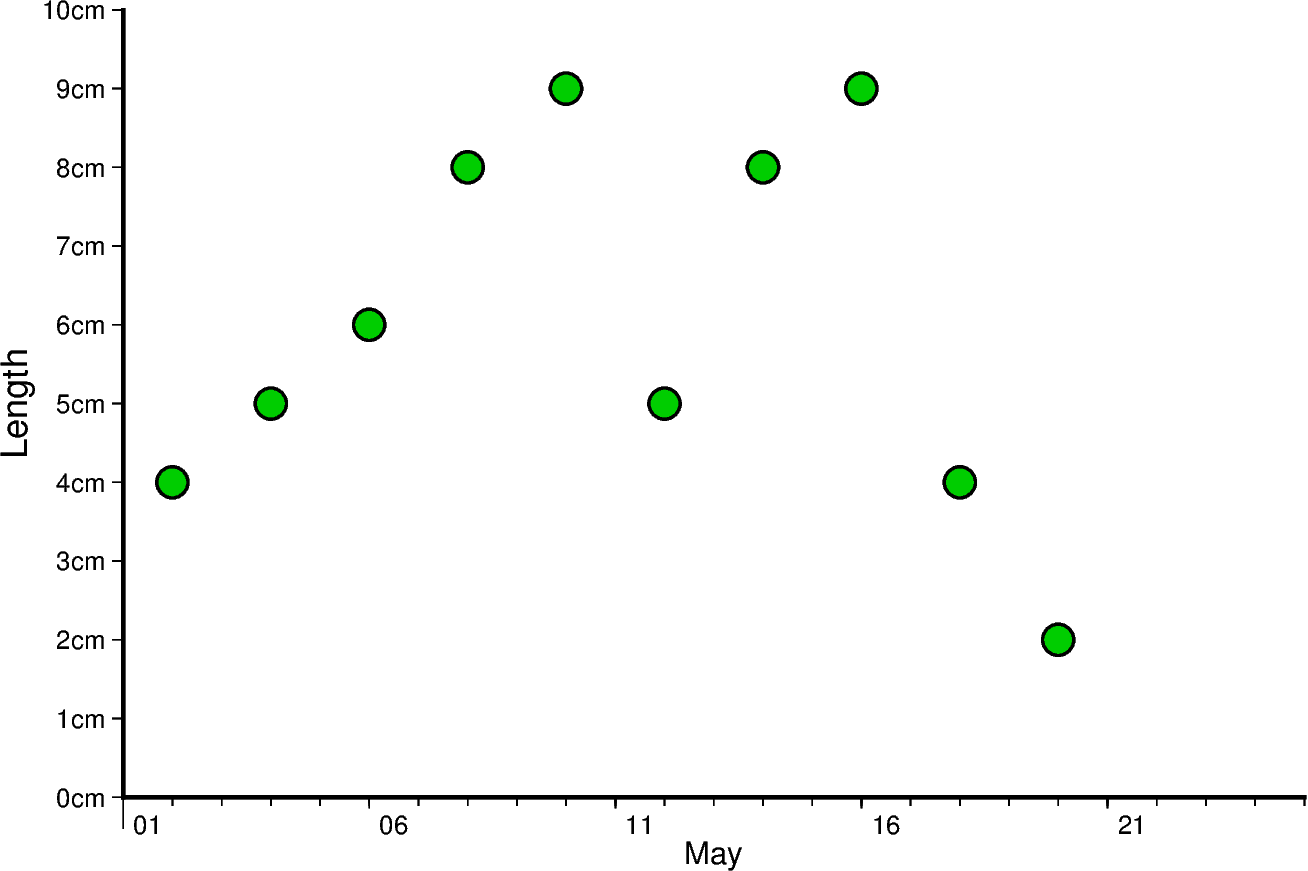Out:

```<IPython.core.display.Image object>
```

The same concept shown above can be applied to smaller as well as larger intervals. In this example, data is plotted for different times throughout two days. Primary x-axis labels are modified to repeat every 6 hours and secondary x-axis label repeats every day and shows the day of the week.

Another notable mention in this example is setting FORMAT_CLOCK_MAP to “-hhAM” which specifies the format used for time. In this case, leading zeros are removed using (-), and only hours are displayed. Additionally, an AM/PM system is being used instead of a 24-hour system. More information about configuring time formats can be found on the official GMT documentation page.

```x = pd.date_range("2021-04-15", periods=8, freq="6H")
y = [2, 5, 3, 1, 5, 7, 9, 6]

fig = pygmt.Figure()
with pygmt.config(FORMAT_CLOCK_MAP="-hhAM"):
fig.plot(
projection="X15c/10c",
region=[
datetime.datetime(2021, 4, 14, 23, 0, 0),
datetime.datetime(2021, 4, 17),
0,
10,
],
frame=["WS", "sxa1K", "pxa6H", "sy+lSpeed", "pya1+ukm/h"],
x=x,
y=y,
style="n0.4c",
pen="1p",
color="lightseagreen",
)
fig.show()
```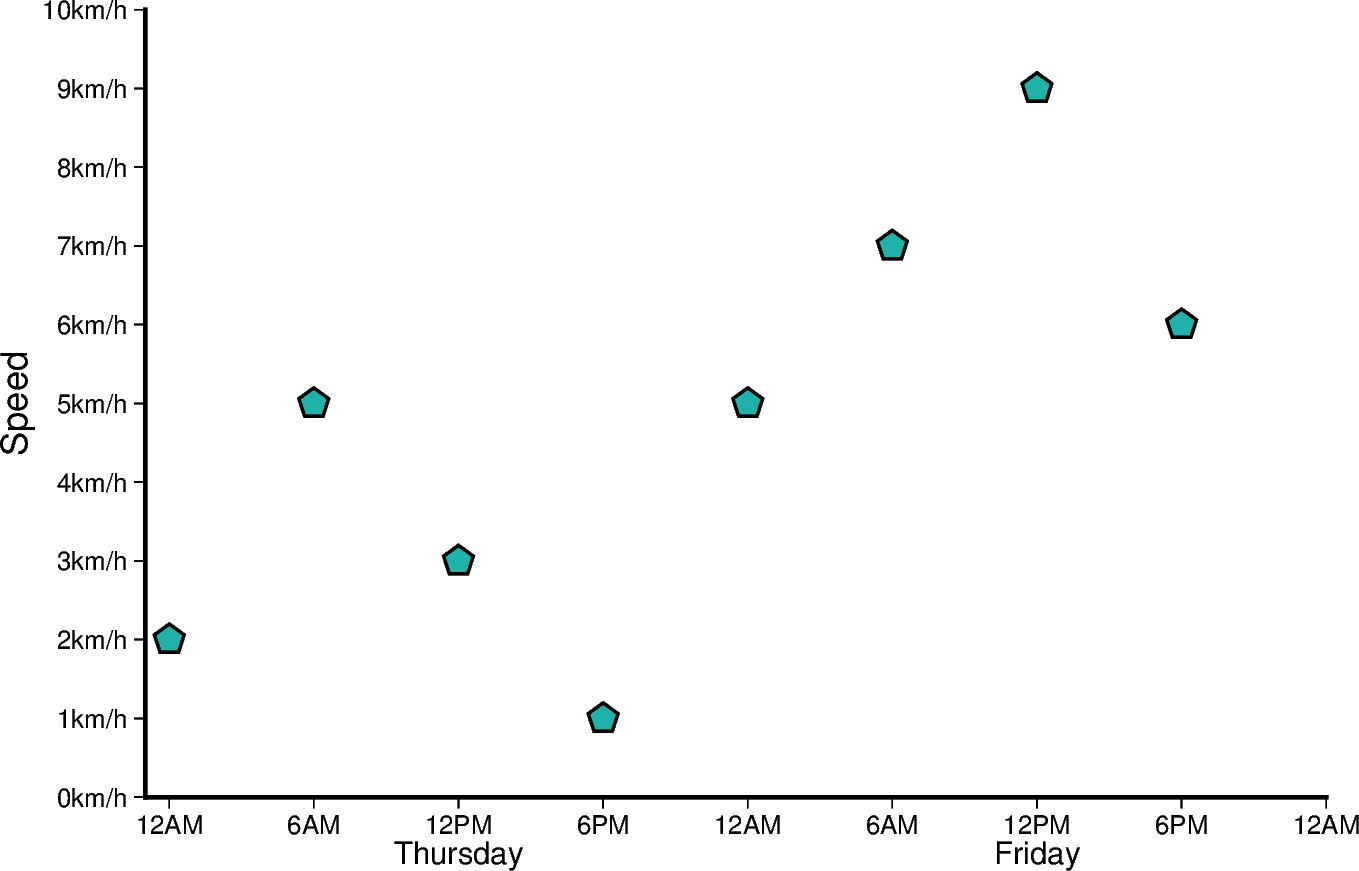Out:

```<IPython.core.display.Image object>
```

Total running time of the script: ( 0 minutes 10.075 seconds)

Gallery generated by Sphinx-Gallery